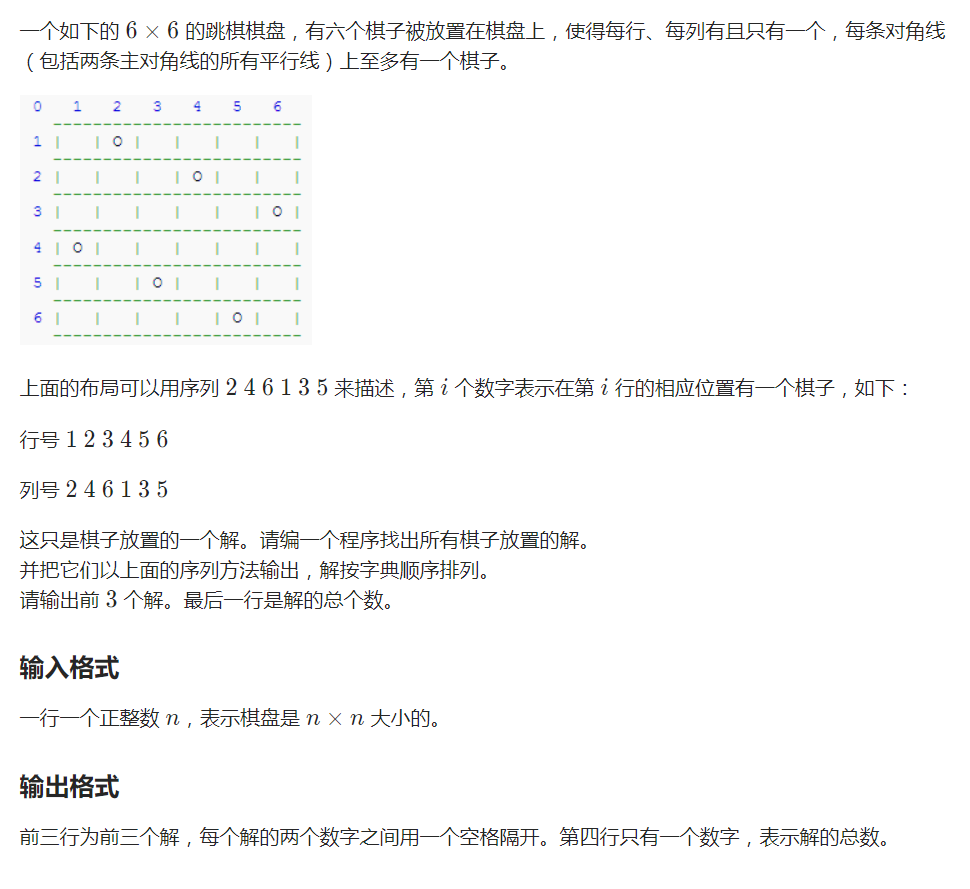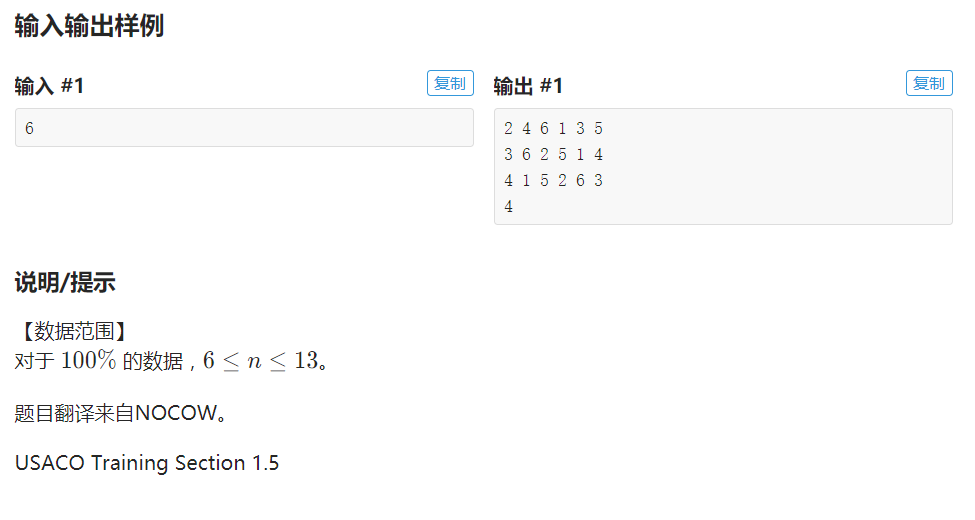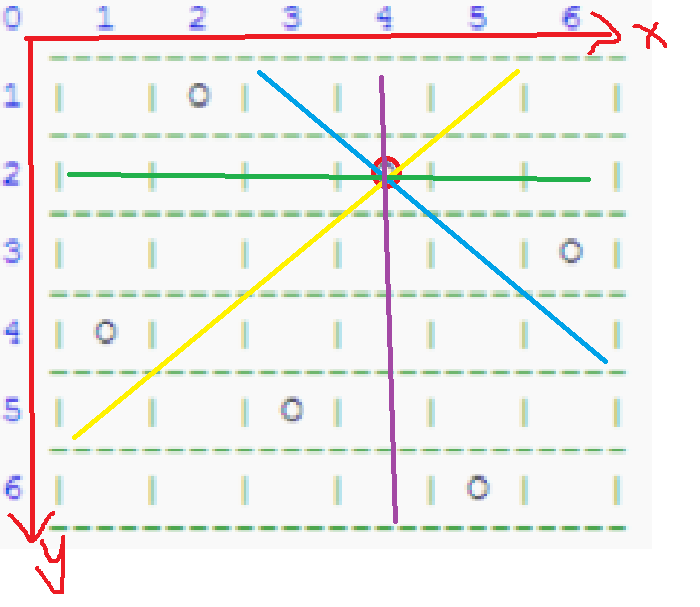P1219 八皇后（dfs+思维）6的格子；1.对于向右斜的黄线，它每个格子上的x坐标+y坐标是一个定值；所以平行于黄线的线上面也有同样的规律；
2.对于向左斜的蓝线，它每个格子上的x坐标-y坐标是一个定值；所以平行于蓝线的线上面也有同样的规律；
3.每行，每列最多放1个皇后，那么我就可以通过枚举每列是放还是不放皇后，然后在dfs里面深度搜索对应的列，然后确定这个位置合法之后才放；

AC代码：

#include<bits/stdc++.h>
using namespace std;
int n;
int r,ma,sa;//分别用来判断是否行合法，主对角是否合法，负对角是否合法
int ans;//记录排列答案
int t;
void dfs(int queen){

//这里参数为什么是queen是因为这里的queen就相当与x坐标，因为每一列都必须有一个皇后，所以参数传这里
if(queen==n+1){

if(t<3){

for(int i=1;i<=n;i++)printf("%d%c",ans[i],i==n?'\n':' ');//按照dfs求全排列可以知道前三个就是字典序最小的，所以没有必要求出总的排列然后再去求字典序最小的
}
t++;
return ;
}
for(int y=1;y<=n;y++){

if(!r[y]&&!ma[queen+y]&&!sa[queen-y+n]){

//第y行可以放并且主对角，副对角也可以放
r[y]=ma[queen+y]=sa[queen-y+n]=1;//标记已经放过了
ans[queen]=y;//在queen列上面的y行放一个
dfs(queen+1);//判断下一行是否能够放
r[y]=ma[queen+y]=sa[queen-y+n]=0;//撤销标记
}
}
}
int main(){

scanf("%d",&n);
dfs(1);//从第一列开始
printf("%d\n",t);
return 0;
}